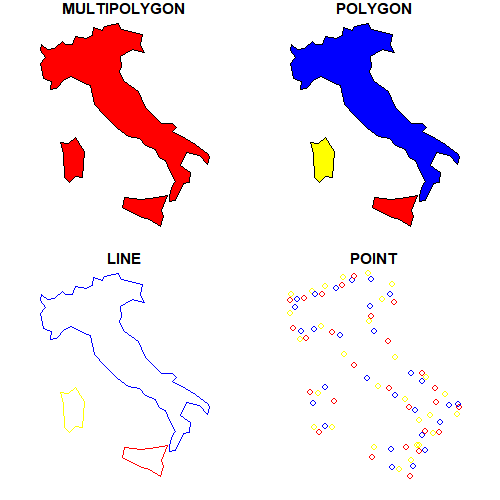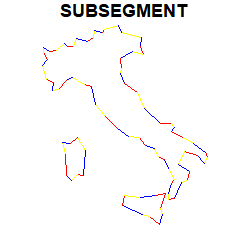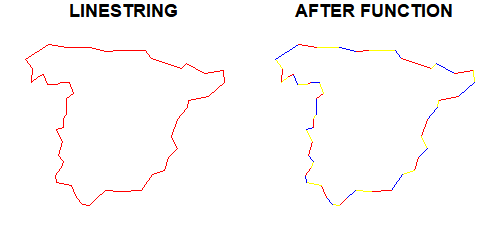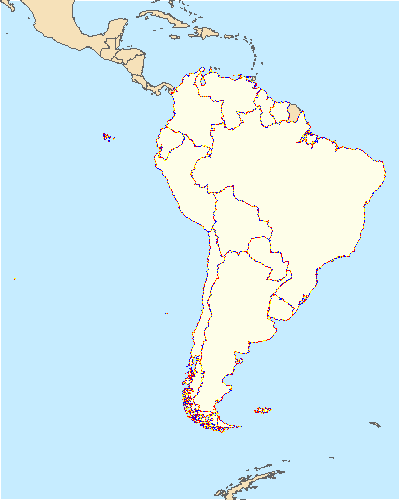# Cast a line to subsegments in R

This post introduces a used-defined function used for casting `sf` objects of `class` `LINESTRING` or `POLYGON` into sub-strings.

## Required R packages

``````library(sf)
library(rnaturalearth)
library(dplyr)``````

## The problem

The `sf`package includes `st_cast`, a very powerful function that transforms geometries into other different types of geometries (i.e. `LINESTRING`to `POLYGON`, etc.).

``````italy <- ne_countries(country = "italy", returnclass = "sf")
italy_pol <- italy %>% st_cast("POLYGON")
italy_lin <- italy_pol %>% st_cast("LINESTRING")
italy_pt <- italy_lin %>% st_cast("POINT")
par(mfrow = c(2, 2), mar = c(1, 1, 1, 1))
plot(st_geometry(italy), col = c("red", "yellow", "blue"), main = "MULTIPOLYGON")
plot(st_geometry(italy_pol), col = c("red", "yellow", "blue"), main = "POLYGON")
plot(st_geometry(italy_lin), col = c("red", "yellow", "blue"), main = "LINE")
plot(st_geometry(italy_pt), col = c("red", "yellow", "blue"), main = "POINT")``````What I missed when using `st_cast`is the possibility to "break" the `LINESTRING`objects into sub-segments:## An approach

So one possible solution could be to create `LINESTRING` objects for each consecutive pair of `POINT`objects across the original geometry. Let's check it:

``````par(mfrow = c(1, 2), mar = c(1, 1, 1, 1))
test <- ne_countries(country = "spain", returnclass = "sf") %>%
st_cast("POLYGON") %>%
st_cast("LINESTRING")
plot(st_geometry(test), col = c("red", "yellow", "blue"), main = "LINESTRING")

geom <- lapply(
1:(length(st_coordinates(test)[, 1]) - 1),
function(i)
rbind(
as.numeric(st_coordinates(test)[i, 1:2]),
as.numeric(st_coordinates(test)[i + 1, 1:2])
)
) %>%
st_multilinestring() %>%
st_sfc(crs = st_crs(test)) %>%
st_cast("LINESTRING")
plot(st_geometry(geom), col = c("red", "yellow", "blue"), main = "AFTER FUNCTION")``````## The function `stdh_cast_substring`

Finally, I wrapped the solution into a function and extended it a little bit:

• When the input is not a `LINESTRING` or a `POLYGON`returns an error and stops.

• The function accepts `sf` with several rows or `sfc` objects with several geometries, and returns the same class of input. In the case of `sf` objects, the input `data.frame` is added.

• By default, the output is a `MULTILINESTRING` geometry. This has the benefit that output has the same number of geometries than the input. This can be modified setting the parameter `to` as `LINESTRING`, that in fact only casts the `MULTILINESTRING`object into `LINESTRING`.

``````stdh_cast_substring <- function(x, to = "MULTILINESTRING") {
ggg <- st_geometry(x)

if (!unique(st_geometry_type(ggg)) %in% c("POLYGON", "LINESTRING")) {
stop("Input should be  LINESTRING or POLYGON")
}
for (k in 1:length(st_geometry(ggg))) {
sub <- ggg[k]
geom <- lapply(
1:(length(st_coordinates(sub)[, 1]) - 1),
function(i)
rbind(
as.numeric(st_coordinates(sub)[i, 1:2]),
as.numeric(st_coordinates(sub)[i + 1, 1:2])
)
) %>%
st_multilinestring() %>%
st_sfc()

if (k == 1) {
endgeom <- geom
}
else {
endgeom <- rbind(endgeom, geom)
}
}
endgeom <- endgeom %>% st_sfc(crs = st_crs(x))
if (class(x) == "sf") {
endgeom <- st_set_geometry(x, endgeom)
}

if (to == "LINESTRING") {
endgeom <- endgeom %>% st_cast("LINESTRING")
}
return(endgeom)
}``````

The function could be improved in terms of performance. Given that it works at a coordinate level, for high-resolution objects it has some degree of delay

``````test100 <- ne_countries(
continent = "south america",
returnclass = "sf"
) %>%
st_cast("POLYGON")

test50 <- ne_countries(50,
continent = "south america",
returnclass = "sf"
) %>%
st_cast("POLYGON")

init <- Sys.time()
t1 <- stdh_cast_substring(test100, "LINESTRING")
end <- Sys.time()
kable(end - init, format = "markdown")``````
x
0.2212591 secs
``````init <- Sys.time()
t2 <- stdh_cast_substring(test50, "LINESTRING")
end <- Sys.time()
kable(end - init, format = "markdown")``````
x
2.996987 secs
``````par(mfrow = c(1, 1), mar = c(0, 0, 0, 0))
plot(st_geometry(test50), col = NA, bg = "#C6ECFF")
plot(st_geometry(ne_countries(50, returnclass = "sf")), col = "#F6E1B9", border = "#646464", add = T)
plot(st_geometry(test50), col = "#FEFEE9", border = "#646464", add = T)
plot(st_geometry(t2), col = c("red", "yellow", "blue"), add = T, lwd = 0.5)``````It can be seen a difference in terms of performance, noting that `test100` has 15 polygons decomposed in 914 sub-strings while `test50` has 80 polygons to 8,414 sub-strings. In that sense, the original `st_cast`is much faster, although this solution may work well in most cases.

A collection of my user-defined functions can be checked here